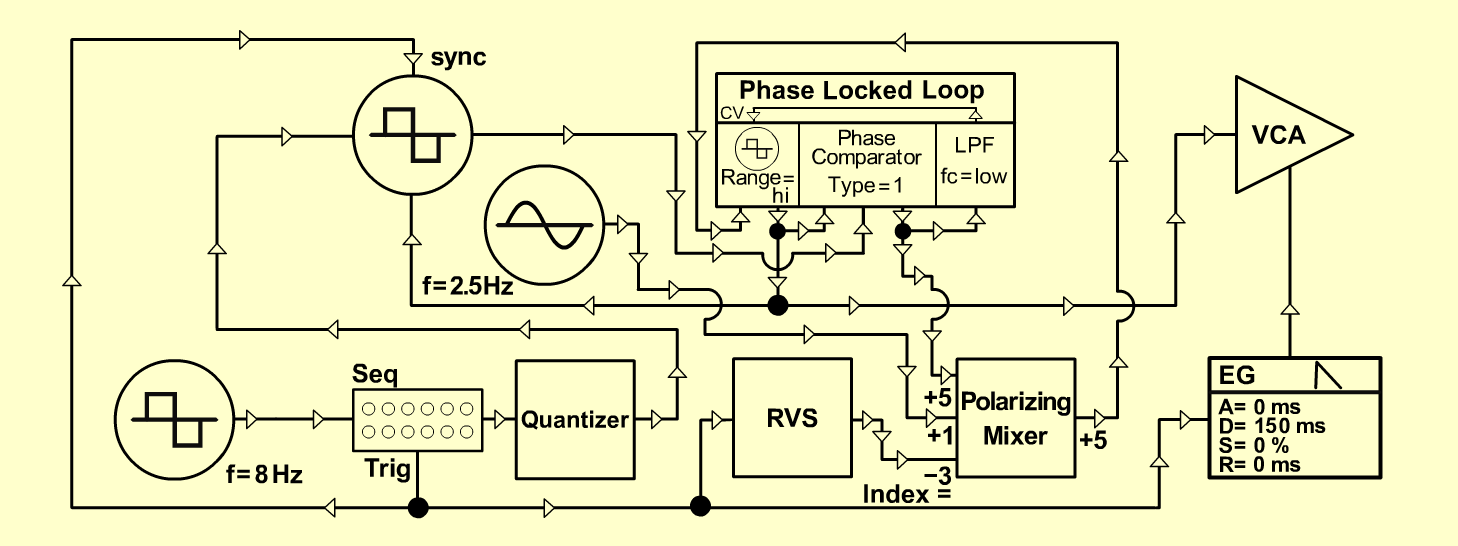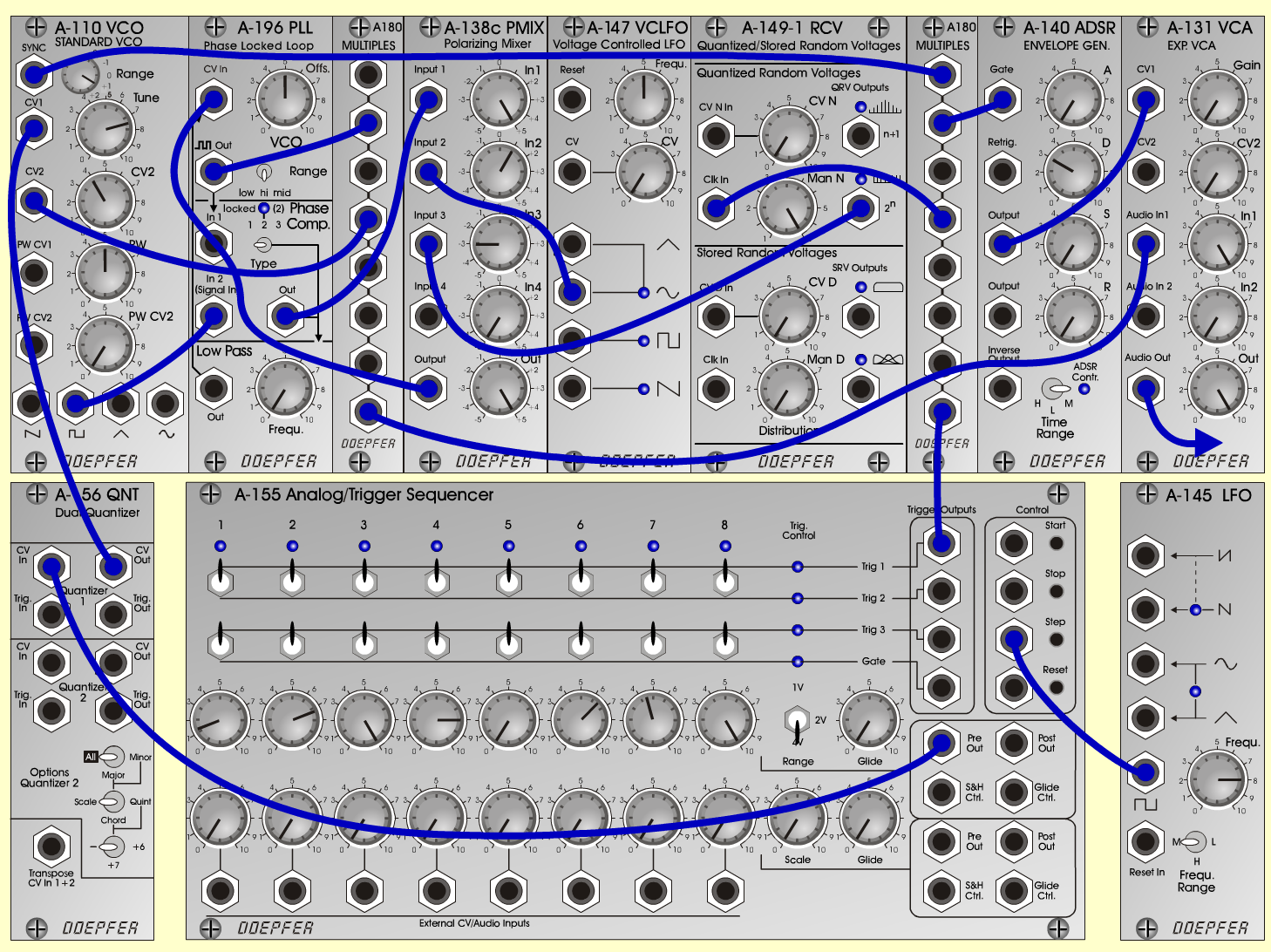PLL-Rnd-FMExperimentalD o e p f e r A - 100 Connections: Settings: A-145 (Square) <=> A-155 (Step/Clock)   A-155 (Trig 1 Out) <=> A-180/1   A-155 (Pre Out) <=> A-156 (CV In)   A-180/1 <=> A-140 (Gate)   A-180/1 <=> A-149-1 (QRV Clk In)   A-180/1 <=> A-110 (SYNC)   A-156-1 (CV Out) <=> A-110 (CV 1)   A-110 (Square) <=> A-196 (In 2)   A-196 (VCO Out) <=> A-180/2   A-180/2 <=> A-110 (CV 2)   A-180/2 <=> A-131 (Audio In)   A-196 (Phase Comp. Out) <=> A-138c (Input 1)   A-147 (Sine) <=> A-138c (Input 2)   A-149-1 (QRV 2n) <=> A-138c (Input 3)   A-138c (Output) <=> A-196 (CV In)   A-131 (Audio Out) <=> Amplifier A-145 (Frq = 8, Range = M)   A-155 (Row Up: 1 = 1.3, 2 = 7.3, 3 = 1  0,   4 = 8, 5 = 0, 6 = 6.5, 7 = 4.5, 8 = 10,   Range = 4V, Glide = 0)   A-110 (CV 2 = 4, PW = 5)   A-196 (Offs. = 5, Range = hi, Type = 1,   Frequ. = 0)   A-147 (Freq = 5)   149-1 (Man N = 6)   A-138c (In 1 = +5, In 2 = +1, In 3 = -3, Out = +5)   A-140 (A = 0, D = 3, S = 0, R = 0, Range = M)   A-131 (Gain = 0, Audio In 1 = 10, Audio Out = 10) Ingo Zobel, June 2005  www.selfoscillate.de  *Sound sample PLL-Rnd-FM